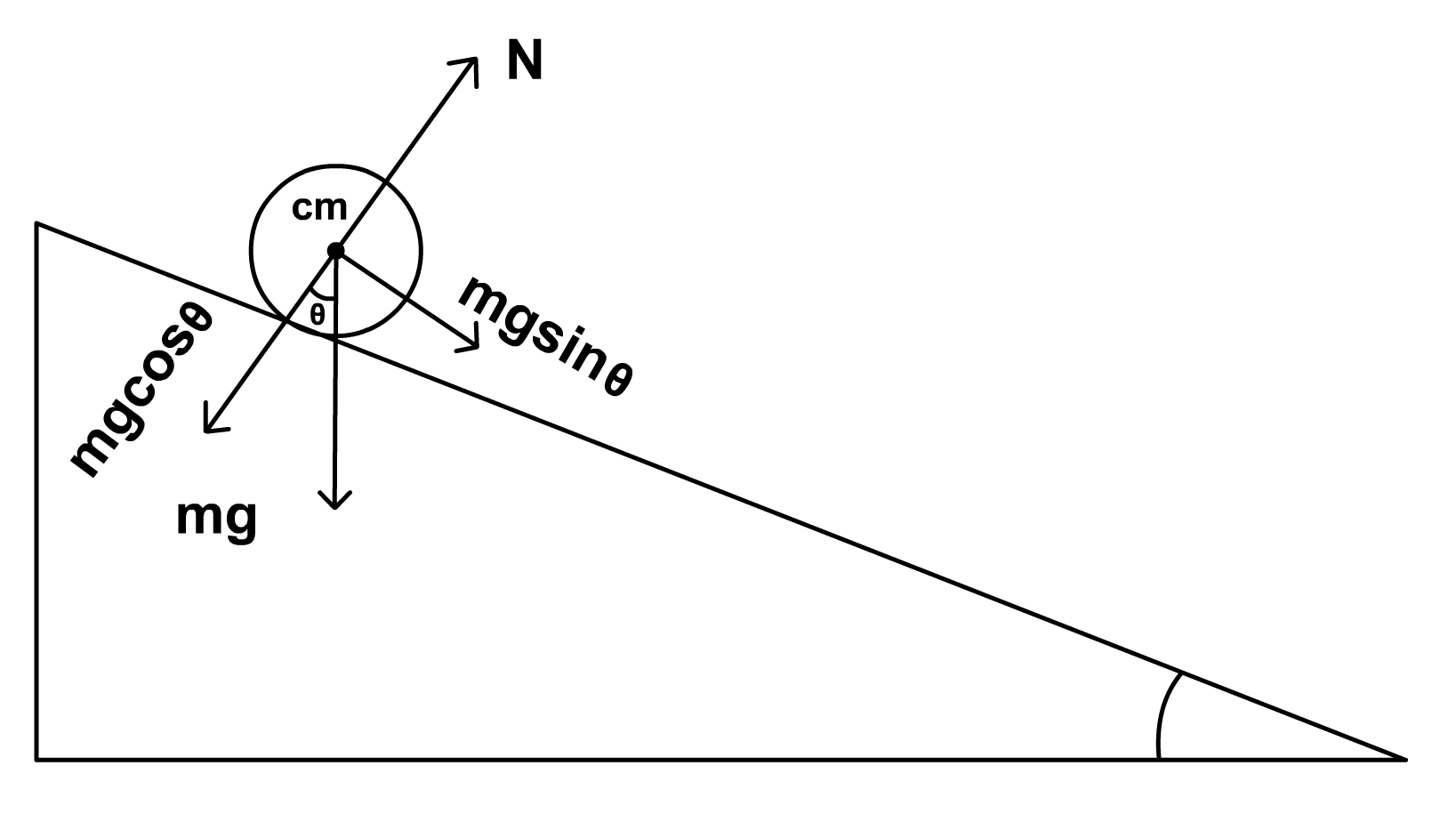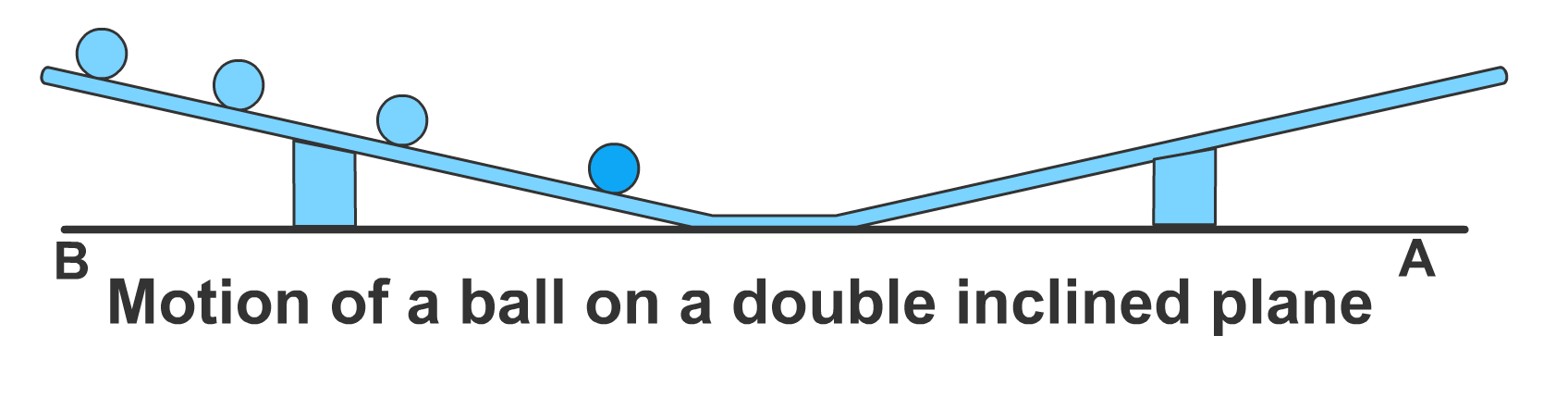Motion of a Ball on an Inclined Track

# Our Objective

To demonstrate the nature of motion of a ball on an inclined track.

# The Theory

An inclined plane, commonly referred to as a ramp, is a flat supporting surface tilted at an angle, with one end higher than the other. Objects placed on an inclined plane will slide down the surface with acceleration due to the unbalanced force acting on it.

Consider a ball on a friction-free inclined surface, tilted at an angle θ. Such a ball will be acted upon by two different forces, namely gravitational force, and normal force. The gravitational force is experienced by all objects with mass. It always acts vertically downward. The normal force, on the other hand, acts perpendicular to the surface on which the object is placed. Here both the forces involved are not acting along the same line. Therefore, to simplify and comprehend the motion, we can resolve the components of gravitational force given by F= mg, such that one component of it acts along the inclined plane (mg sin θ) while the other component acts in the direction opposite to the normal force (mg cos θ). Observe the free-body diagram given below. The mg cos θ component balances the normal force while the mg sin θ component of force provides the necessary acceleration (g sin θ) for the object to roll down the surface. The acceleration of the object will remain constant throughout the motion.Now let us assume there is friction on the surface. The frictional force will oppose the motion and act opposite to the sine component of force of gravity.Therefore, the net force acting on the ball along the inclination, F = m g sin θ – fs

Here, the frictional force f = μ mg cos θ

where μ is the coefficient of friction along the path.

The force down the inclined plane must therefore be greater than the frictional force for the object to slide down the surface. As the friction along the path increases, the force acting along the incline decreases. Similarly, the extent to which the inclined plane is tilted will alter the acceleration that an object will experience when sliding down the plane. While the gravitational force acting on the ball does not change depending on the angle of inclination, a steeper incline will give a larger component force that is pushing the ball down the ramp. This is because sinθ will increase with θ for θ between 0 and (π/2). As there is a greater force pulling the block down the plane, a steeper incline will cause the block to begin descending when it may not have on a shallower incline.

It should be noted that the frictional force always acts at the point where the ball and inclined plane make contact, but the gravitational force always acts at the centre of mass. In the case of a ball, both forces are not acting in the same direction and are separated by a radius, R. This produces torque, which leads to rolling motion.

We can also have a doubly inclined plane as shown in the figure. A ball at rest at the top of an inclined track has only potential energy and zero kinetic energy. As the body rolls down the track, its potential energy gradually gets converted into kinetic energy. It has zero potential energy and maximum kinetic energy at the bottom of the track. If the same ball rolls up the second inclined track, its kinetic energy decreases, and potential energy increases. The ball will continue its motion until all its kinetic energy gets converted to potential energy. The motion stops when the ball attains zero kinetic energy. This will continue as the ball rolls back from the second track. If there is no friction in the track, the total mechanical energy (or the sum of the kinetic and the potential energy of the ball) will remain constant (conserved) throughout the motion of ball and the ball will continue its motion indefinitely.# Learning Outcomes

The students learn to :

• Describe the forces acting on a ball moving on an inclined track.
• Resolve component of force.
• Identify the unbalanced force that produces the acceleration.
• Analyze rolling motion using energy conservation.